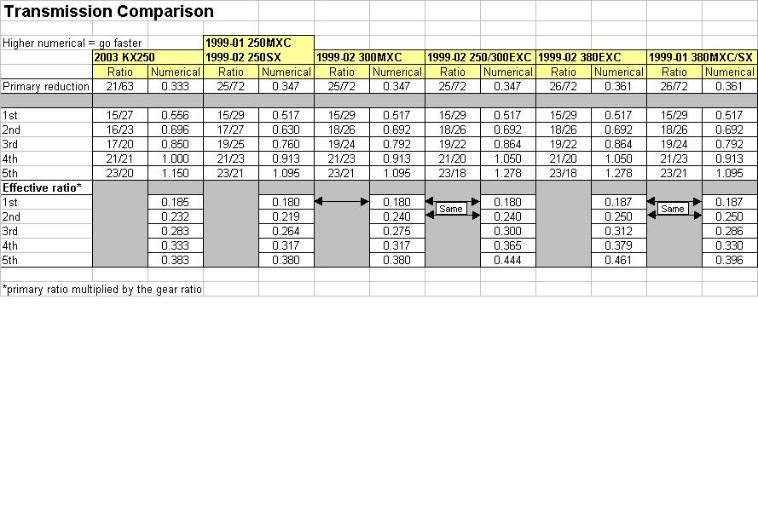Ever wondered how your transmission compares to other
bikes? Gearheads like me spend sleepless nights pondering
these things, and gearheads with a lot of time on their hands,
well, let's just say Excel tables can get created. The table
below summarizes transmission ratios from a sampling of
KTM's from 1999-02 and my 2003 KX250.

The comparison starts with the primary drive, which is the
gear connected to the cranskshaft. The table below shows
that most of the bikes are initially geared down to about 1/3
of crankshaft speed.  From there, further "gearing down"
takes place at the main shaft and countershaft.

In the table below, the numerator in the Primary Reduction
ratio is the number of teeth on the sprocket attached to the
crankshaft. The denominator is the number of teeth on the
clutch sprocket. In the individual gear ratios, the numerator of
the ratio is the number of teeth on the sprocket attached to
the main shaft (same shaft on which the clutch spins) which
corresponds to what gear you're in. The denominator is the
number of teeth on the countershaft (same shaft that spins
the countershaft sprocket), again, corresponding to what gear
you're in.
Transmission ComparisonsSo how do these transmission variations affect how fast your
bike will go? It's pretty simple, really. Multiply the primary
gear reduction by the appropriate main/countershaft gear
ratio, then multiply that number by the ratio of the number of
teeth on the countershaft sprocket to the number of teeth on
the rear sprocket. Still with me? Good. Now take that number
and multiply it by the engine RPM's to get the RPM's at the
wheel. Figure out the distance (in feet) your wheel travels in
one revolution, multiply that number by the rear wheel RPM's
to get distance traveled per minute, then multiply that
number by 60 to get the distance traveled in an hour. That
number is feet traveled per hour, so divide by 5,280 to get
miles traveled per hour.

Simple.

But even simpler is using my handy spreadsheet to let the
computer do most of the work. All you have to come up with
is the number of teeth on the appropriate gears inside your
transmission.

Want to try out the spreadsheet?

Keep in mind that there are inefficiencies in turning engine
RPM's into ground speed, so don't expect your bike to go
quite as fast as what the spreadsheet calculates. But it
provides a relative idea of what gear ratios and sprocket
combinations do for overall speed.